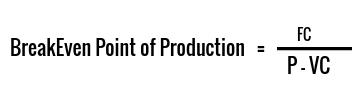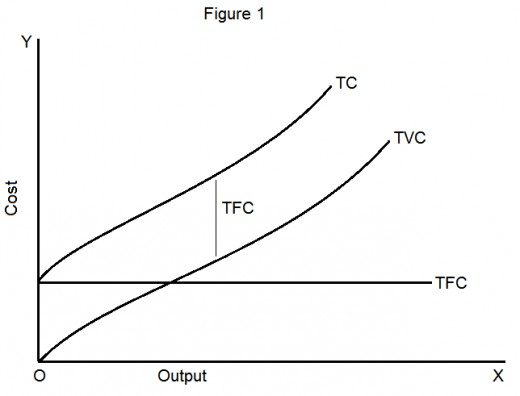# Break-Even Analysis

The break-even point can be defined as the production and sales levels of a given product at which the revenue generated from the sales is perfectly equal to the production cost.
• The Breakeven point is the point at which the company breaks even, i.e it does not make any profit/loss.
• At Breakeven Point: Total Cost of Production (TC) = Total Revenue from Sales (TR).
• Total Cost of Production: Total cost of production consists of two types of costs :
1. Total Fixed Cost (TFC)
2. Total Variable Cost (TVC)

### Fixed Cost:

• It is the summation of all expenses that do not change as the level of production varies. It remains the same regardless of the level of production of any company. It does not change with the number of units produced.
• E.g: Rent, Electricity Bill, Interest Rate Charged, etc
• Example: Company XYZ is involved in the production of tires. It rents a warehouse for the same. Now even if no tire is produced in a particular month, the company has to pay the rent of the warehouse. So the rent will be considered as Fixed Charge.

### Variable Cost:

• It is the summation of all variable expenses. Variable Costs are directly proportional to the volume of goods produced. The more the goods produced, the more is the variable cost and vice versa.
• E.g: Cost of Raw Material, Wages paid to laborers, etc.
• Example: Company XYZ is involved in the production of bread from wheat flour. 100 bread packets production requires 2 kg of wheat. Now if the company produces 1000 bread packets in a month, it will incur a cost of 20 kg of wheat. Also, if the company changes its production to 2000 bread packets, it will incur a cost of 40 kg of wheat. Here, the cost of raw material i.e cost of wheat is an example of Variable Cost.

#### Profit of any firm will be given as :

• Total Profit = Total Revenue from sales (TR) - Total cost of production(TC)

#### At Break-Even point, there is no profit and no loss, so:

• Total Profit = 0
• Total Revenue from sales (TR) - Total cost of production(TC) = 0
• Total Revenue from sales (TR) = Total cost of production (TC)
• Total Revenue from sales (TR) = Total Fixed Cost + Total Variable Cost
• If n is the number of units produced for Breakeven :
• (n) * ( Revenue from Sales/unit) = Fixed Cost + (n) * (Variable Cost/unit)
• n = Fixed Cost /(Revenue from sales/unit - Variable Cost/unit)

## At Breakeven point,Where, FC = Fixed Cost
P = Revenue from sales per unit
VC = Variable Cost incurred per unit

#### Example:

The total fixed cost of a manufacturing company is Rs 4,50,000/- and the variable cost per unit produced is Rs 150/- and the selling price of one unit is Rs 300/-. Calculate the break-even point of production.
Solution :BEP = 4,50,000/(300-150)
BEP = 3000

#### Example:

The total fixed cost of a balloon company is Rs 2,00,000/-. If the company has to produce 2000 balloons for breakeven and revenue from sales is Rs Rs 8,00,000/-, calculate the variable cost per unit.
Solution :Now , Revenue from sales/unit = 8,00,000/2,000 = Rs 400
Let the Variable Cost/unit be Rs X.
2000 = 2,00,000/ (400 - X)
400 - X = 100
X = Rs 300
Hence, the variable cost per unit is Rs 300.

## The Cost Curve is given as follows :Here,
• Total Fixed Cost (TFC) does not change with units produced i.e, Output.
• Total Variable Cost (TVC) increases as the number of units produced increases.
• Total Cost (TC ) = TFC + TVC, also increases with the number of units produced.
• The gap between TC and TVC i.e, (TC-TVC) equals TFC (Total Fixed Cost).